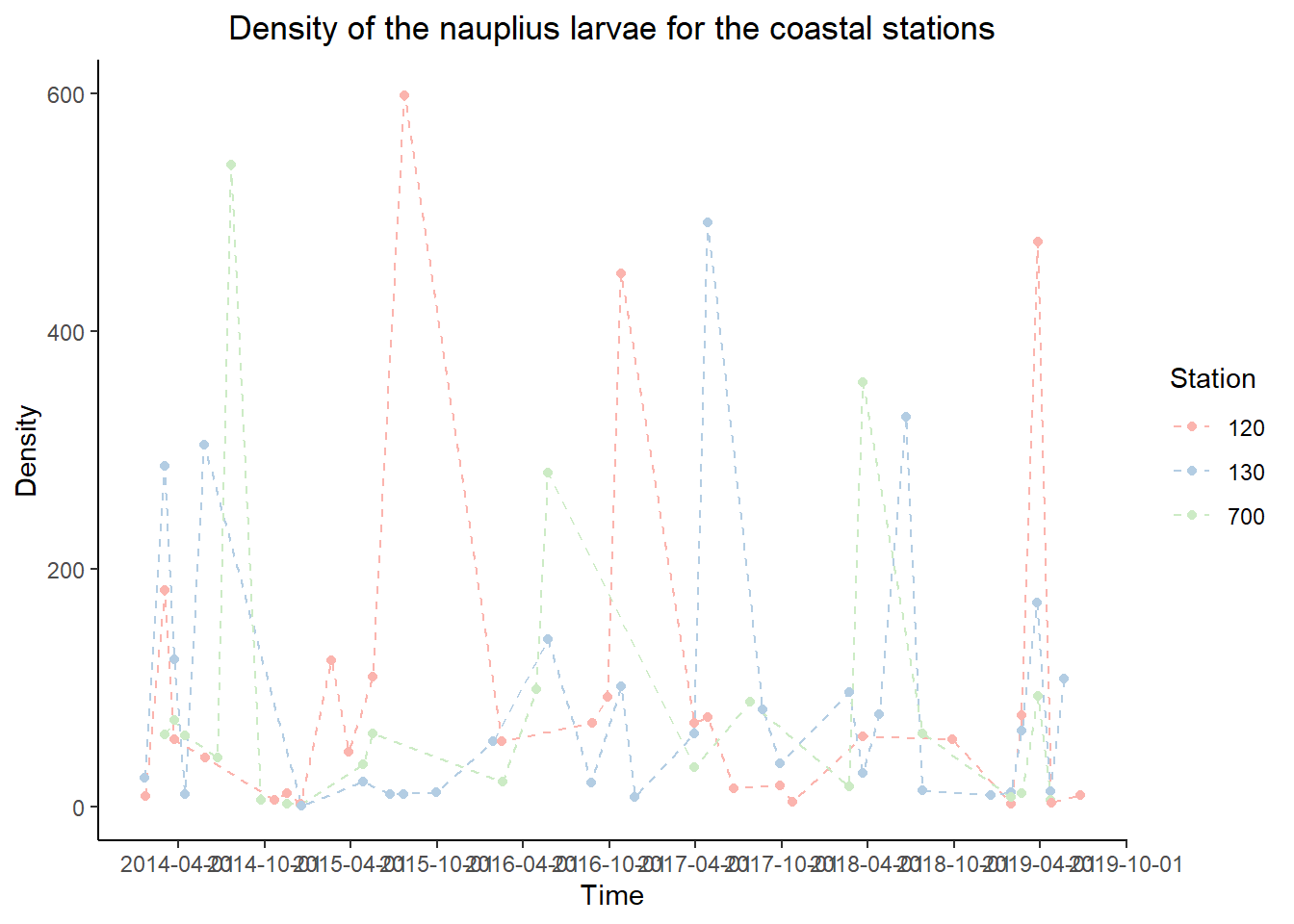Contributors: Anaïs Aubert (Flanders Marine Insitute) & Nanou Goedefroo (Flanders Marine Institute)
Source data: LifeWatch Zooplankton data explorer (rshiny.lifewatch.be/zooscan-data)
Institute: LifeWatch (www.lifewatch.be)

This code makes use of the zooplankton data sampled by LifeWatch. Time series are calculated and visualized of the larval stages of important biofouling species in the Belgian Part of the North Sea (BPNS). Bivalve, cirriped and gastropod larvae can be visualized in time for the different stations in the BPNS.

library(dplyr)
library(data.table)
library(lubridate)
library(ggplot2)
library(gridExtra)
library(scales)

ZooIn=read.table("Zooplankton.csv",sep=";", header=TRUE)
View(ZooIn)

Or the zooplankton can be read in via the LW R package (Link). A connection to the VLIZ server is needed to install the LW data package on your local computer.

#library(lwdata)
#ZooIn<- getZooscanData(startdate = "2014-01-20", stopdate = Sys.Date())

## 2. Data preparation

The ‘Time’ variable now is a character. In order to change this to a date (POSIXct format), we use the dmy_hm function (similar to the time format in the LifeWatch data files)

ZooIn$Time <- dmy_hm(ZooIn$Time)

To visualize the stations seperately, these are subsetted

stations <- ZooIn %>%
split(.$Station) Stations 120, 130 and 700 are closely located to the coast and are therefore considered as coastal stations. coastal <- rbind(stations$"120", stations$"130", stations$"700")
coastal <- aggregate(coastal$Density, by=list(coastal$Station,coastal$Taxon,coastal$Time),
FUN=mean,
na.rm=TRUE)
names <- c("Station", "Taxon", "Time","Density")
colnames(coastal) <- names

The other stations: 710, 780, W07BIS, 435 are located more offshore.

offshore <- rbind(stations$"710", stations$"780", stations$"435", stations$W07BIS)
offshore <- aggregate(offshore$Density, by=list(offshore$Station,offshore$Taxon,offshore$Time),
FUN=mean,
na.rm=TRUE)

colnames(offshore) <- names

Further subsetting can be done for the different groups. Here we will subset the nauplius larvae and the cypris larvae of the cirripedes for the coastal and offshore subsets. Currently bivalve and gastropod larvae have no seperate group but are grouped as molusks (‘Mollusca’) in the dataset.

coastal <- data.table(coastal)
coastal.taxa <- coastal %>%
split(.$Taxon) offshore <- data.table(offshore) offshore.taxa <- offshore %>% split(.$Taxon)

station.taxa <- ZooIn %>%
split(list(.$Station, .$Taxon))

## 3. Plot the zooplankton data: Time series

Some example plots are presented and can be adapted by entering different data - and subsets.

Plot the density of nauplius larvae for the different coastal stations in time

plot.coastal.nauplius <- ggplot(data = coastal.taxa\$Cirripeda_naup,
aes(x = Time, y = Density)) +
geom_line(aes(color = Station),
linetype = "dashed") +
geom_point(aes(color = Station)) +
labs(title = 'Density of the nauplius larvae for the coastal stations') +
theme_classic() +
theme(plot.title = element_text(hjust = 0.5)) +
scale_x_datetime(breaks = date_breaks("6 months")) +
scale_color_manual(values = c("#fbb4ae","#b3cde3","#ccebc5"))
plot.coastal.nauplius